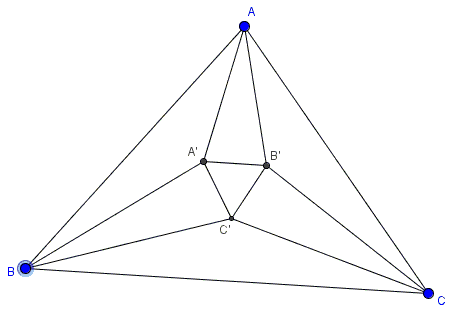# Morley's Theorem: A Proof That Needs Fixing

Morley's theorem asserts that in the diagram below $\Delta A'B'C'$ is equilateral, whatever $\Delta ABC.$The proof below is taken verbatim from a recent book on problem-solving.

Let $\angle BAC=3\alpha,$ $\angle ABC=3\beta,$ $\angle ACB=3\gamma.$ Let $B',$ $T,$ $S$ be the points of intersection of the trisectors.If we assume that $2\alpha +2\gamma\le 60^{\circ},$ $2\alpha +2\beta\le 60^{\circ},$ and $2\beta +2\gamma\le 60^{\circ}$ then

$4\alpha +4\beta+4\gamma\le 180^{\circ},$

i.e., $\alpha +\beta+\gamma\le 45^{\circ},$ which implies $3\alpha +3\beta+3\gamma\le 135^{\circ},$ or, equivalently, $180^{\circ}\le 135^{\circ},$ a contradiction. We conclude that one of the sums $2\alpha +2\gamma,$ $2\alpha +2\beta,$ $2\beta +2\gamma$ should exceed $60^{\circ}.$ Suppose $2\alpha +2\gamma\gt 60^{\circ},$ then $\angle ABC\lt 90^{\circ}.$

Let $B'B_{1}\perp AC$ and $B'B_{3}\perp AT.$ Then, since every point of the angle bisector is equidistant from both sides of the angle, we get

$B'B_{3}=2B'D=2B'B_{1}.$

Similarly, if we consider $B'B_{2}\perp CS,$ we obtain $B'B_{2}=2B'B_{1},$ and thus

$B'B_{2}=B'B_{3}.$

We also observe that $\angle B_3B'B_{2}=2\alpha+2\gamma\gt 60^{\circ}.$

Consider the points $A',$ $C'$ on the semi-straight lines $AT$ and $CS,$ respectively, so that triangle $A'B'C'$ is isosceles. We obviously have$\angle A'B'B_{3}=\angle C'B'B_{2}=s,$

therefore

$2s=2\alpha +2\gamma - 60^{\circ}.$

Observe that $A'B_{3}=A'B'$ (due to symmetry) and $C'B_{2}=C'B'.$ It follows that

$s=\alpha +\gamma - 30^{\circ}$

and

$2h+2\alpha +2\gamma=180^{\circ},$

hence $h=\angle B_{2}B{3}B'=\angle B'B_{2}B_{3}.$ Consequently, we have $h=90^{\circ}-\alpha -\gamma,$ thus

\begin{align} h - s &= 120^{\circ}-2\alpha -2\gamma\\ &= 120^{\circ}-\frac{2}{3}(3\alpha +3\gamma)\\ &= 120^{\circ}-\frac{2}{3}(180^{\circ}-3\beta)\\ &= 2\beta, \end{align}

and thus $u=2b,$ where $u=\angle B_{2}B_{3}A'=\angle SB_{2}B_{3}.$ At this point, we observe that

$B_{2}A'=A'C'=C'B_{2}$

because of the isosceles triangle $A'B'C',$ and thus

\begin{align} \angle B_{3}C'B_{2} &= 180^{\circ}-u-\frac{u}{2}\\ &= 180^{\circ}-\frac{3u}{2}\\ &= 180^{\circ}-3\beta. \end{align}

It follows that the quadrilateral $B_{3}C'B_{2}$ is inscribed in a circle, and similarly, we obtain that the quadrilateral $BB_{2}A'B_{3}$ can be inscribed in a circle, as well. Consequently the straight lines $BA',$ $BC'$ trisect $\angle ABC,$ hence $T=A'$ and $S=C'.$

### References

1. S. E. Louridas, M. Th. Rassias, Problem-Solving and Selected Topics in Euclidean Geometry, Springer, 2013 (65-68)### Morley's Miracle

#### Invalid proofs

1. Bankoff's conundrum
2. Proof by Nolan L Aljaddou
3. Morley's Theorem: A Proof That Needs Fixing